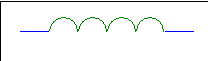# Inductance

There are three circuit elements that exist and can be used to model all electronic devices. Previously, we met resistance the first circuit element and the only one responsible for energy dissipation (as heat). Inductors are the second circuit element. They have the property of storing energy in magnetic fields.

Physically inductors are nothing more than coils. Cylindrical (solonoidal) and doughnut shaped (toroidal) geometries are most common.

Symbol :Self Inductance, L
The self inductance of a coil may be defined as the ratio of the magnetic flux linking the coil (due to current flowing in the coil) to the current flowing in the coil.

Mathematically, L = φ/I

where L = inductance of coil measured in Henries (H)
I = current flowing through coil in Amperes (A)
φ = magnetic flux linking the coil in Webers (Wb)

The self inductance of a coil may also be defined as the ratio of its back-emf to the current flowing in the coil.

### Energy stored in a Magnetic Field

The current flowing through a coil sustains a magnetic field around the coil. The energy stored in this field is independent of time and is given by the following equation.

W = ½LI2

where L = inductance in Henries (H)
I = current in Amperes (A)
W = energy in Joules (J)

#### Worked Examples

Example 2.6.1

Calculate the energy stored in the B field of an inductor of value 4 H when 3 A flows through it.

Solution: W = ½LI2

W = 0.5 × 4 × 32 = 18 J

Example 2.6.2

Calculate the inductance of a coil that stores 27 J in its B field when 3 A flows through it.

Solution: W = ½LI2

Transposing, L = 2W/I2

L = 2×27/32 = 6 H